# Unit 1.7

8 Sep 2014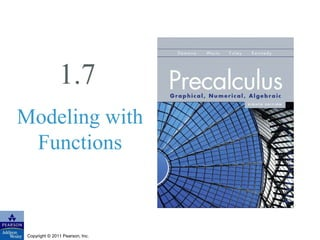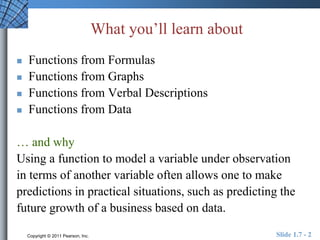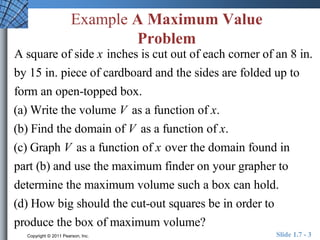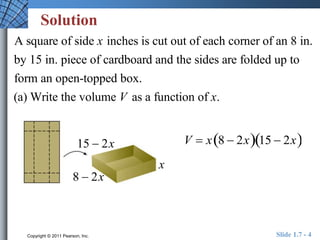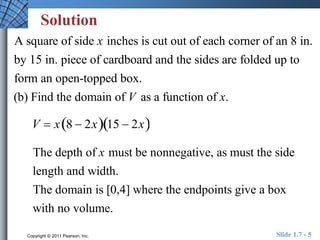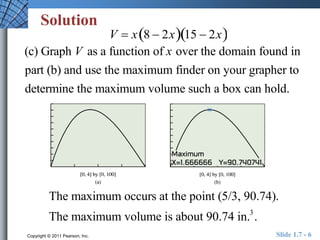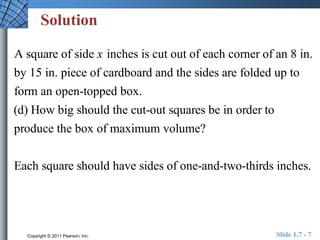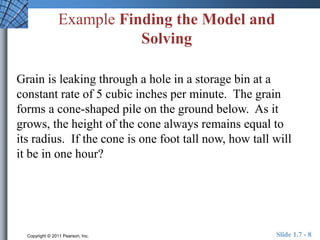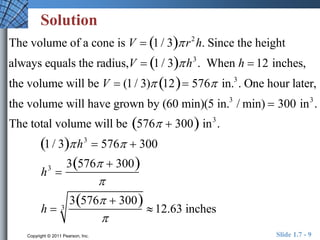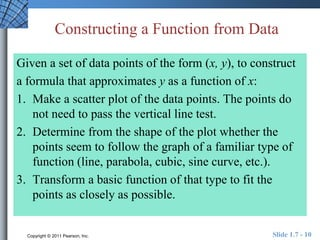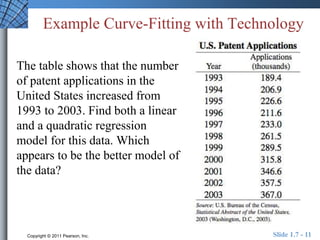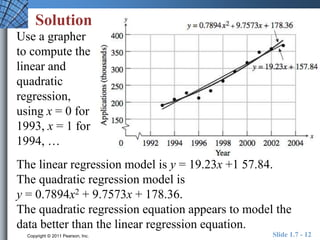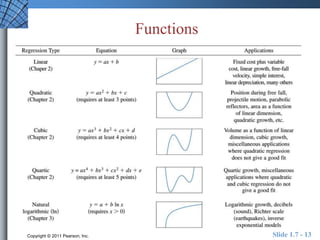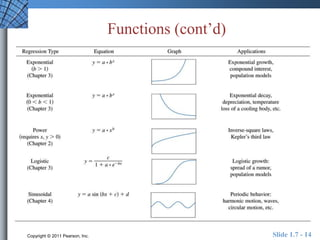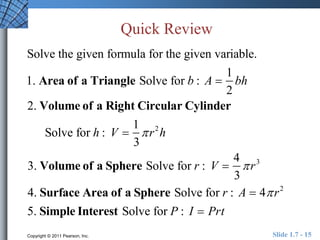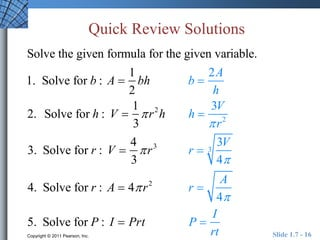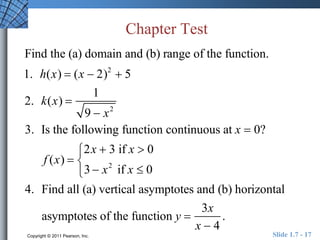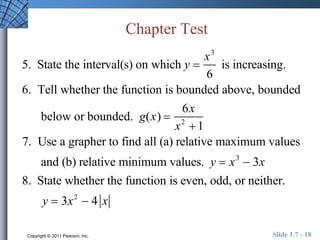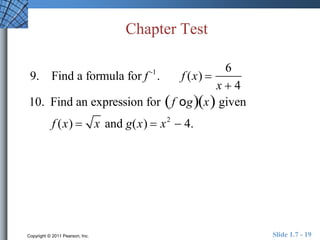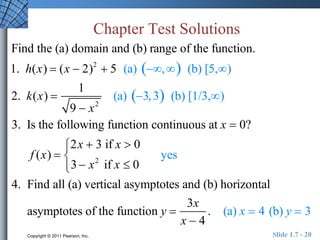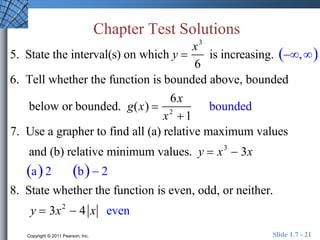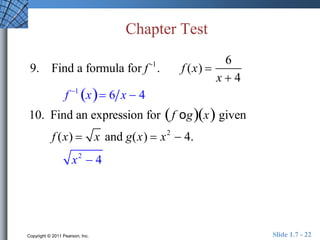1 sur 22

### Unit 1.7

• 2. What you’ll learn about  Functions from Formulas  Functions from Graphs  Functions from Verbal Descriptions  Functions from Data … and why Using a function to model a variable under observation in terms of another variable often allows one to make predictions in practical situations, such as predicting the future growth of a business based on data. Copyright © 2011 Pearson, Inc. Slide 1.7 - 2
• 3. Example A Maximum Value Problem A square of side x inches is cut out of each corner of an 8 in. by 15 in. piece of cardboard and the sides are folded up to form an open-topped box. (a) Write the volume V as a function of x. (b) Find the domain of V as a function of x. (c) Graph V as a function of x over the domain found in part (b) and use the maximum finder on your grapher to determine the maximum volume such a box can hold. (d) How big should the cut-out squares be in order to produce the box of maximum volume? Copyright © 2011 Pearson, Inc. Slide 1.7 - 3
• 4. Solution A square of side x inches is cut out of each corner of an 8 in. by 15 in. piece of cardboard and the sides are folded up to form an open-topped box. (a) Write the volume V as a function of x. 15  2x V  x8  2x15  2x x 8  2x Copyright © 2011 Pearson, Inc. Slide 1.7 - 4
• 5. Solution A square of side x inches is cut out of each corner of an 8 in. by 15 in. piece of cardboard and the sides are folded up to form an open-topped box. (b) Find the domain of V as a function of x. V  x8  2x15  2x The depth of x must be nonnegative, as must the side length and width. The domain is [0,4] where the endpoints give a box with no volume. Copyright © 2011 Pearson, Inc. Slide 1.7 - 5
• 6. Solution V  x8  2x15  2x (c) Graph V as a function of x over the domain found in part (b) and use the maximum finder on your grapher to determine the maximum volume such a box can hold. The maximum occurs at the point (5/3, 90.74). The maximum volume is about 90.74 in.3. Copyright © 2011 Pearson, Inc. Slide 1.7 - 6
• 7. Solution A square of side x inches is cut out of each corner of an 8 in. by 15 in. piece of cardboard and the sides are folded up to form an open-topped box. (d) How big should the cut-out squares be in order to produce the box of maximum volume? Each square should have sides of one-and-two-thirds inches. Copyright © 2011 Pearson, Inc. Slide 1.7 - 7
• 8. Example Finding the Model and Solving Grain is leaking through a hole in a storage bin at a constant rate of 5 cubic inches per minute. The grain forms a cone-shaped pile on the ground below. As it grows, the height of the cone always remains equal to its radius. If the cone is one foot tall now, how tall will it be in one hour? Copyright © 2011 Pearson, Inc. Slide 1.7 - 8
• 9. Solution The volume of a cone is V  1 / 3 r2h. Since the height always equals the radius,V  1 / 3 h3 . When h  12 inches, the volume will be V  (1 / 3) 12 576 in.3 . One hour later, the volume will have grown by (60 min)(5 in.3 / min)  300 in3 . The total volume will be 576  300 in3 . 1 / 3 h3  576  300 h3  3576  300  h  3576  300 3  12.63 inches  Copyright © 2011 Pearson, Inc. Slide 1.7 - 9
• 10. Constructing a Function from Data Given a set of data points of the form (x, y), to construct a formula that approximates y as a function of x: 1. Make a scatter plot of the data points. The points do not need to pass the vertical line test. 2. Determine from the shape of the plot whether the points seem to follow the graph of a familiar type of function (line, parabola, cubic, sine curve, etc.). 3. Transform a basic function of that type to fit the points as closely as possible. Copyright © 2011 Pearson, Inc. Slide 1.7 - 10
• 11. Example Curve-Fitting with Technology The table shows that the number of patent applications in the United States increased from 1993 to 2003. Find both a linear and a quadratic regression model for this data. Which appears to be the better model of the data? Copyright © 2011 Pearson, Inc. Slide 1.7 - 11
• 12. Solution Use a grapher to compute the linear and quadratic regression, using x = 0 for 1993, x = 1 for 1994, … The linear regression model is y = 19.23x +1 57.84. The quadratic regression model is y = 0.7894x2 + 9.7573x + 178.36. The quadratic regression equation appears to model the data better than the linear regression equation. Copyright © 2011 Pearson, Inc. Slide 1.7 - 12
• 13. Functions Copyright © 2011 Pearson, Inc. Slide 1.7 - 13
• 14. Functions (cont’d) Copyright © 2011 Pearson, Inc. Slide 1.7 - 14
• 15. Quick Review Solve the given formula for the given variable. 1. Area of a Triangle Solve for b : A  1 2 bh 2. Volume of a Right Circular Cylinder Solve for h : V  1 3  r2h 3. Volume of a Sphere Solve for r : V  4 3  r3 4. Surface Area of a Sphere Solve for r : A  4 r2 5. Simple Interest Solve for P : I  Prt Copyright © 2011 Pearson, Inc. Slide 1.7 - 15
• 16. Quick Review Solutions Solve the given formula for the given variable. 1. Solve for b : A  1 2 bh b  2A h 2. Solve for h : V  1 3  r2h h  3V  r2 3. Solve for r : V  4 3  r3 r  3V 4 3 4. Solve for r : A  4 r2 r  A 4 5. Solve for P : I  Prt P  I rt Copyright © 2011 Pearson, Inc. Slide 1.7 - 16
• 17. Chapter Test Find the (a) domain and (b) range of the function. 1. h(x)  (x  2)2  5 2. k(x)  1 9  x2 3. Is the following function continuous at x  0? f (x)  2x  3 if x  0 3  x2 if x  0  4. Find all (a) vertical asymptotes and (b) horizontal asymptotes of the function y  3x x  4 . Copyright © 2011 Pearson, Inc. Slide 1.7 - 17
• 18. Chapter Test 5. State the interval(s) on which y  x3 6 is increasing. 6. Tell whether the function is bounded above, bounded below or bounded. g(x)  6x x2  1 7. Use a grapher to find all (a) relative maximum values and (b) relative minimum values. y  x3  3x 8. State whether the function is even, odd, or neither. y  3x2  4 x Copyright © 2011 Pearson, Inc. Slide 1.7 - 18
• 19. Chapter Test 9. Find a formula for f -1. f (x)  6 x  4 10. Find an expression for  f ogx given f (x)  x and g(x)  x2  4. Copyright © 2011 Pearson, Inc. Slide 1.7 - 19
• 20. Chapter Test Solutions Find the (a) domain and (b) range of the function. 1. h(x)  (x  2)2  5 (a) , (b) [5,) 2. k(x)  1 9  x2 (a) 3, 3 (b) [1/3,) 3. Is the following function continuous at x  0? f (x)  2x  3 if x  0 3  x2 if x  0  yes 4. Find all (a) vertical asymptotes and (b) horizontal asymptotes of the function y  3x x  4 . (a) x  4 (b) y  3 Copyright © 2011 Pearson, Inc. Slide 1.7 - 20
• 21. Chapter Test Solutions 5. State the interval(s) on which y  x3 6 is increasing. , 6. Tell whether the function is bounded above, bounded below or bounded. g(x)  6x x2  1 bounded 7. Use a grapher to find all (a) relative maximum values and (b) relative minimum values. y  x3  3x a 2 b  2 8. State whether the function is even, odd, or neither. y  3x2  4 x even Copyright © 2011 Pearson, Inc. Slide 1.7 - 21
• 22. Chapter Test 9. Find a formula for f -1. f (x)  6 x  4 f 1 x 6 x  4 10. Find an expression for  f ogx given f (x)  x and g(x)  x2  4. x2  4 Copyright © 2011 Pearson, Inc. Slide 1.7 - 22